## The seventh week of machine learning-logistic regression of binary classification algorithm

1. Logistic classification algorithm

Logistic regression (LR) is a classification model in traditional machine learning. Due to the advantages of simple and efficient algorithms, good interpretability, and easy expansion, it is widely used in click-through rate estimation (CTR) and computational advertising ( CA) and recommendation system (RS) and other tasks. Although the name of logistic regression is regression, it is actually a classification learning method. Mainly used for two classification problems, using the Logistic function (or Sigmoid function), the value range of the independent variable is (-INF, INF), the value range of the independent variable is (0,1), the function form is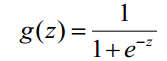Because the definition domain of sigmoid function is (-INF, + INF), and the value domain is (0, 1). Therefore, the most basic LR classifier is suitable for classifying two classification (class 0, class 1) targets. The Sigmoid function is a beautiful "S" shape, as shown below: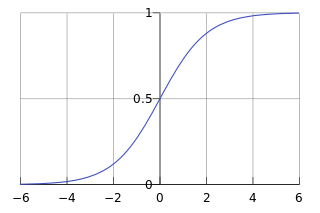The purpose of the LR classifier is to learn a 0/1 classification model from the training data features. This model takes the linear combination of sample features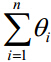as independent variables and uses the logistic function to map the independent variables to (0, 1). Therefore, the solution of the LR classifier is to solve a set of weights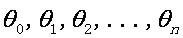( Θ 0 is a nominal variable-dummy, which is a constant, and often x0 = 1.0 in actual engineering. Regardless of the meaning of the constant term, it is best to keep it), and substitute the Logistic function Construct a prediction function: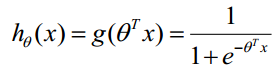The value of the function represents the probability that the result is 1, which is the probability that the feature belongs to y = 1. Therefore, the probability that the input x classification results are category 1 and category 0 are: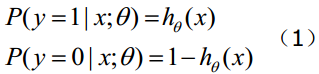When we want to determine which class a new feature belongs to, find a z value according to the following formula:,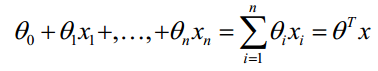(x1, x2, ..., xn are each feature of a certain sample data, the dimension is n). Then find out-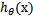if it is greater than 0.5, it is the category of y = 1, otherwise it belongs to the category of y = 0. (Note: It is still assumed that the statistical samples are evenly distributed, so the threshold is set to 0.5). How can the weights of the LR classifier be obtained? This requires the concepts of maximum likelihood estimation MLE and optimization algorithms. The most commonly used optimization algorithms in mathematics are gradient ascent (descending) algorithms. Logistic regression can and can be used for multiple classifications, but the two classifications are more commonly used and easier to explain. So the most commonly used in practice is the logistic regression of binary classification. LR classifier is applicable to data types: numeric and nominal data. Its advantage is that the calculation cost is not high, and it is easy to understand and implement; its disadvantage is that it is easy to underfit and the classification accuracy may not be high.

Second, gradient descent to solve the loss function

The loss function of logistic regression is derived from the maximum likelihood estimation of the coefficients in the logistic regression itself, and the maximum likelihood estimation is to inversely infer the parameters that lead to the result by the known result through the known result. Maximum likelihood estimation is the application of probability theory in statistics. It provides a method for evaluating model parameters given observation data, that is, "the model is fixed, the parameters are unknown". Through several experiments, the results are observed. Using the experimental results to obtain a certain parameter value can maximize the probability of sample appearance, which is called maximum likelihood estimation. Logistic regression is a kind of supervised learning. It has a training label and has a known result. Starting from this known result, we can derive the parameter that can obtain the maximum probability. As long as we have this parameter, our The model can naturally predict the unknown data very accurately.

Let the model of logistic regression be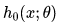that it can be regarded as the posterior probability of class 1, so there are: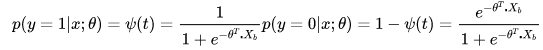The above two formulas can be rewritten into the general form: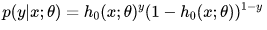Therefore, according to the maximum likelihood estimation, we can get: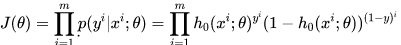To simplify the calculation, taking the logarithm will give: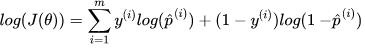We hope that the greater the maximum likelihood, the better, that is, for a given number of samples m, the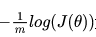smaller the hope, the better. The loss function of logistic regression is as follows: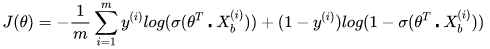Next, you need to find a set of parameters so that the above loss function reaches the minimum value. There is no standard equation solution to this loss function, so in actual optimization, we often use the gradient descent method to continuously approximate the optimal solution.

Using the gradient descent method, the gradient is required, and for each parameter in each vector , the corresponding derivative is obtained: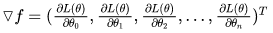Differentiate the sigmoid function (chain derivation rule):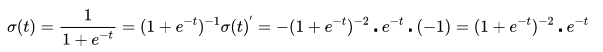Then derive the log function of the outer layer: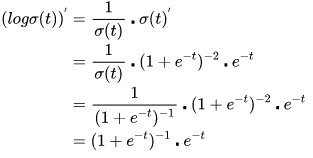Then organize: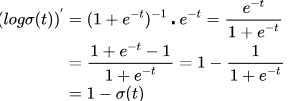Next, the expression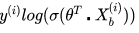of the first half of the loss function can be derived to Θ. Bringing the above result, we get: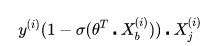Similarly, the second half of the loss function can be differentiated, similar to the above. The derivative of the final loss function L (Θ) to Θ is as follows, that is, the logistic regression loss function derivates a parameter through the gradient descent method, and the result is as follows:Among them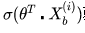is the predicted value of the logistic regression model.

After the derivative of a parameter is obtained, the loss function can be differentiated in all feature dimensions, and the vectorized result is as follows: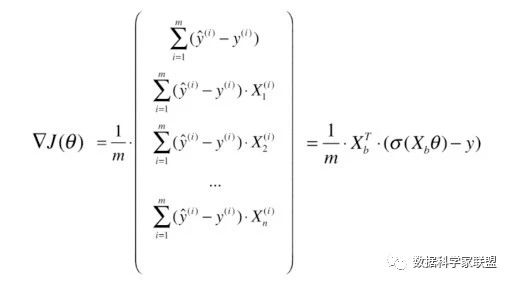Three. Python code implementation

### Guess you like

Origin www.cnblogs.com/mindy-snail/p/12675217.html
Recommended
Ranking
Daily